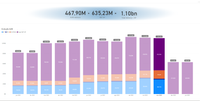cancel
Showing results for
Did you mean:Frequent Visitor

## How to show a measure filtered by last availble data but override when another date is selected.

As you can see I have 3 cards showing SUM of measure from different tables and the last one is a SUM for both:

``Total Advisory = sum(Advisory[AUM])``
``Total NET XP = SUM('Historico Diversificador Web'[NET])``
``Total Advisory + XP = [Total Advisory] + [Total NET XP]``If no date range is selected the cards will show a SUM for the whole period which is not what we want since this is historical evolution data not cumulative.

These data sets are coming from different people and as you can see we still havent received the data for December and January from the Total Advisory table.

What I am trying to do is have the cards show data for the previous available month when nothing on the page is selected and have it show the selection when a chart date is selected.

1 ACCEPTED SOLUTIONCommunity Support

I think your data model should be a DimDate table and a Fact table. DimDate table andFact table is connected by [Date] columns. You can try PREVIOUSMONTH function. Please try below code.

``````Total Advisory =
VAR _CURRENT =
CALCULATE ( SUM ( Advisory[AUM] ) )
VAR _PREVIOUS =
CALCULATE ( SUM ( Advisory[AUM] ), PREVIOUSMONTH ( DimDate[Date] ) )
RETURN
IF ( ISFILTERED ( DimDate[Date] ), _CURRENT, _PREVIOUS )``````
``````Total NET XP = SUM('Historico Diversificador Web'[NET])
VAR _CURRENT =
CALCULATE ( SUM('Historico Diversificador Web'[NET]) )
VAR _PREVIOUS =
CALCULATE ( SUM('Historico Diversificador Web'[NET]), PREVIOUSMONTH ( DimDate[Date] ) )
RETURN
IF ( ISFILTERED ( DimDate[Date] ), _CURRENT, _PREVIOUS )``````
``Total Advisory + XP = [Total Advisory] + [Total NET XP]``

Best Regards,
Rico Zhou

If this post helps, then please consider Accept it as the solution to help the other members find it more quickly.

2 REPLIES 2Community Support

I think your data model should be a DimDate table and a Fact table. DimDate table andFact table is connected by [Date] columns. You can try PREVIOUSMONTH function. Please try below code.

``````Total Advisory =
VAR _CURRENT =
CALCULATE ( SUM ( Advisory[AUM] ) )
VAR _PREVIOUS =
CALCULATE ( SUM ( Advisory[AUM] ), PREVIOUSMONTH ( DimDate[Date] ) )
RETURN
IF ( ISFILTERED ( DimDate[Date] ), _CURRENT, _PREVIOUS )``````
``````Total NET XP = SUM('Historico Diversificador Web'[NET])
VAR _CURRENT =
CALCULATE ( SUM('Historico Diversificador Web'[NET]) )
VAR _PREVIOUS =
CALCULATE ( SUM('Historico Diversificador Web'[NET]), PREVIOUSMONTH ( DimDate[Date] ) )
RETURN
IF ( ISFILTERED ( DimDate[Date] ), _CURRENT, _PREVIOUS )``````
``Total Advisory + XP = [Total Advisory] + [Total NET XP]``

Best Regards,
Rico Zhou

If this post helps, then please consider Accept it as the solution to help the other members find it more quickly.Solution Sage

Hey @diogodasilva,

I'm not exactly sure what your data model looks like and what your tables and columns are called. Perhaps this formula will help you further. You still have to adjust the correct references to tables and columns.

``````Total Advisory =
IF (
ISFILTERED ( 'Table'[YearMonth] ),
VAR _last_month =
LASTNONBLANKVALUE (
'Table'[YearMonthOrder],
CALCULATE ( SUM ( Advisory[AUM] ) )
)
RETURN
CALCULATE ( SUM ( Advisory[AUM] ), 'Table'[YearMonthOrder] = _last_month )
)``````

You can do the same for Total NET XP, only the SUM expression will change.Announcements#### Exclusive opportunity for Women!

Join us for a free, hands-on Microsoft workshop led by women trainers for women where you will learn how to build a Dashboard in a Day!#### Power Platform Conference-Power BI and Fabric Sessions

Join us Oct 1 - 6 in Las Vegas for the Microsoft Power Platform Conference.Top Solution Authors
Top Kudoed Authors
Users online (1,867)